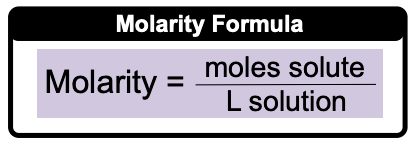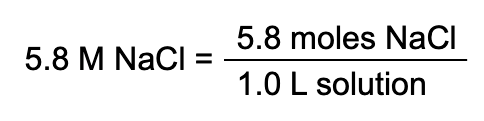Clutch Prep is now a part of Pearson
Ch.4 - Chemical Quantities & Aqueous ReactionsWorksheetSee all chapters

# Molarity

See all sections
Sections
Solutions
Molarity
Osmolarity
Dilutions
Solubility Rules
Electrolytes
Molecular Equations
Gas Evolution Equations
Solution Stoichiometry
Complete Ionic Equations
Calculate Oxidation Numbers
Redox Reactions
Balancing Redox Reactions: Acidic Solutions
Balancing Redox Reactions: Basic Solutions
Activity Series

Ever wonder how a mass amount such as moles can be converted into the volume amount of liters? Well, molarity serves as the bridge between moles and liters.

###### Molarity

Concept #1: Calculate MolarityExample #1: Calculate the molarity of a solution prepared by dissolving 23.7 g NaOH in enough water to make 2.50 L of solution.

Concept #2: Using Molarity to Calculate UnknownsExample #2: How many grams of Na3PO4 (MW: 163.94 g/mol) are present in 300.0 mL of a 0.550 M Na3POsolution?

Practice: What volume in (µL) of 0.125 M HBr contains 0.170 moles HBr?

Practice: Hypernatremia is a medical condition where a patient has high levels of sodium in their blood, and is the result of the body containing too little water. A patient has a measured sodium level of 165 mM. If 30.0 mL of their blood were drawn, what mass (in ng) of sodium would be present?

Practice: 2.64 grams of an unknown compound was dissolved in water to yield 150 mL of solution. The concentration of the solution was 0.075 M. What was the molecular weight of the substance?

Practice: A solution with a final volume of 750.0 mL was prepared by dissolving 30.00 mL of benzene (C6H6, density = 0.8787 g/mL) in dichloromethane. Calculate the molarity of benzene in the solution.